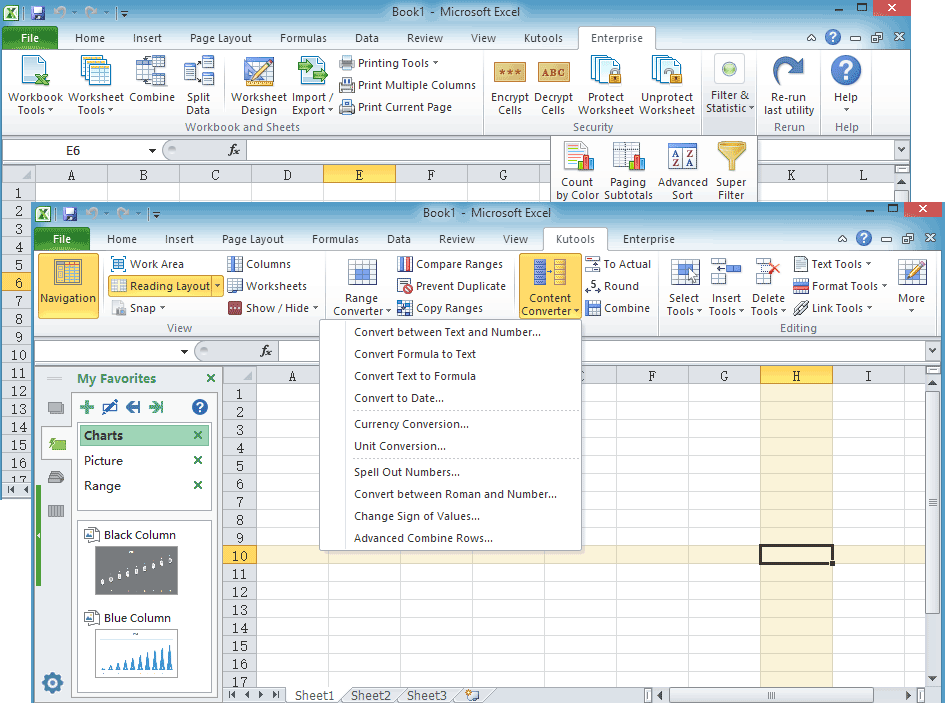Note: The other languages of the website are Google-translated. Back to English

## Quickly apply mathematical operations without using formula in Excel

### Kutools for Excel

We generally use formulas for some common operations in Excel, but using formula in Excel is complex. Kutools for Excel’s Operation tool allows you to easily change values in cells without using formulas. For example, if you want to increase all product prices up 12%, you can quickly change these values in cells without using formula by applying Kutools for Excel’s Operation tools. With this tool, you just need to select all the product prices cells and make them multiply by 112%. You can quickly apply following mathematical operations to a range of cells without using formula:

Add a number to multiple cells or a range of cells

Subtract a number from each cell in a column or a range

Multiply a range of cells by a number

Divide multiple cells or a range of cells by a number

Apply exponential operation to a range of cells

Round a range of numbers to whole numbers or 2 decimal places

Apply a function to a range of cells

Apply formula to multiple or all cells

####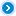Click Kutools >> More >> Operation. See screenshots: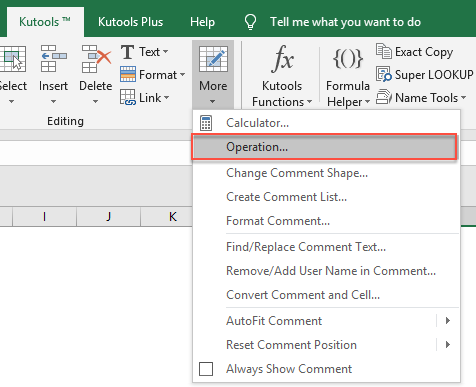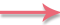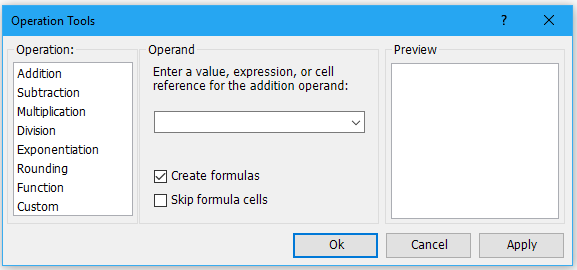####Add a number to multiple cells or a range of cells

If you want to add a number to multiple cells or a range of cells without using a formula in Excel, you can get it done as follows:

1. Select the range that you want to add a number, and then applying the utility by clicking Kutools > More > Operation.

2. In the Operation Tools dialog box, click Addition and then enter the number that you need to add to the range as shown in the below screenshot. And you can see the results from the Preview Pane.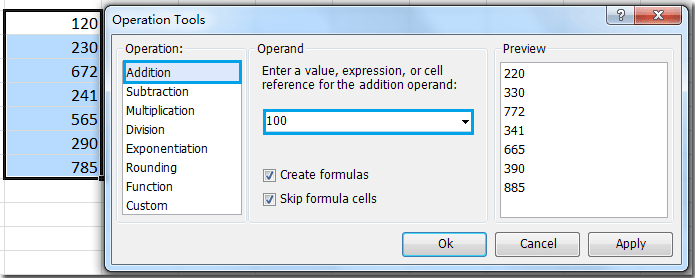3. Then click OK or Apply. The number 100 has been added to the all cells in the range. Note: click OK button will close the dialog box and apply the operation; but click Apply button will only apply the operation without closing the dialog box. See screenshots: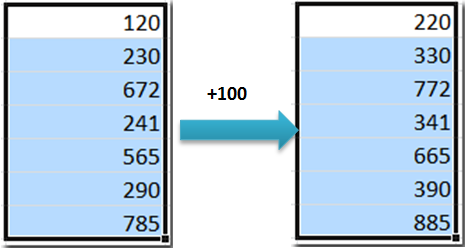####Subtract a number from each cell in a column or a range

If you want to subtract a specific number from each cell in a column or a range without using formula, you can quickly do it with following steps:

1. Select the range that you need to subtract a number from. And then click Subtraction in the Operation Tools dialog box. After entering the number that you need to subtract from the range, such as 95. And you can preview the results from the Preview Pane. See screenshot: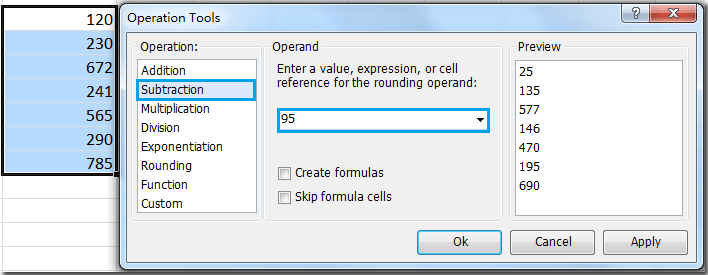2. Then click OK or Apply. It will subtract 95 from the range. See screenshots: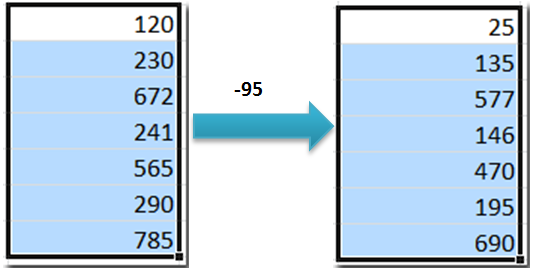####Multiply a range of cells by a number

If you want to multiply a range of cells by a number or percentage without using formula, you can multiply the range by a number as follows:

1. Highlight the range that you want to multiply by a number. Go to the Operation Tools dialog box, click Multiplication from left Operation Pane. Then enter the number that you need to multiply a range of cells by, such as 88%. And you can preview the results from the Preview Pane. See screenshot: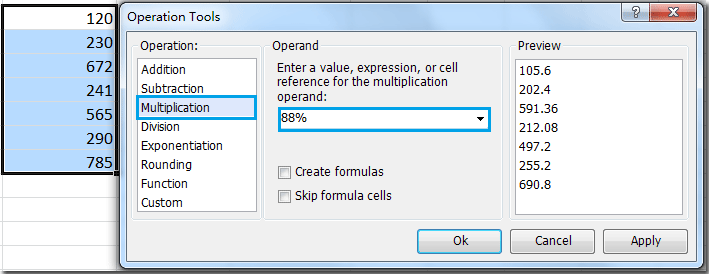2. Then click OK or Apply. The selected cell values have been multiplied by number 88% at once. See screenshots: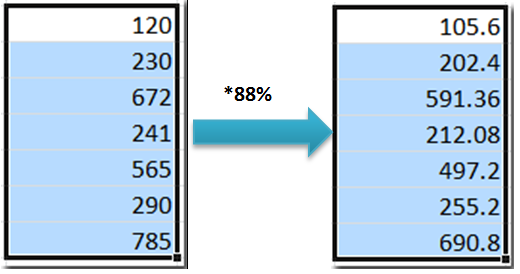####Divide multiple cells or a range of cells by a number

If you want to divide multiple cells or a range of cells by a number without using formula. Do as follows:

1. Highlight the range that you want to divide by a number. Go to the Operation Tools dialog box, click Division from left Operation Pane. Then entering the number that you need to divide the range by, such as 10. And you can preview the results from the Preview Pane. See screenshot: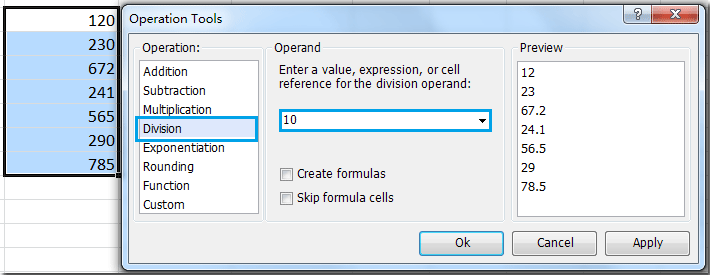2. Then click OK or Apply. The selected cell values have been divided by the number 10 at once. See screenshots: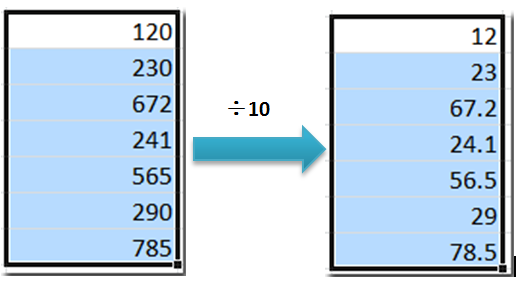####Apply exponential operation to a range of cells

Maybe you don’t know how to do an exponential function in Excel. If you want to apply exponential operation to a range of cell without using formula, how could you do in Excel? You can get it done as follows:

1. Select the range that you want to apply exponential function. In the Operation Tools dialog box, click Exponentiation from left Operation Pane. Then enter the number you need to power for the range cells, such as 2. And you can preview the results from the Preview Pane. See screenshot: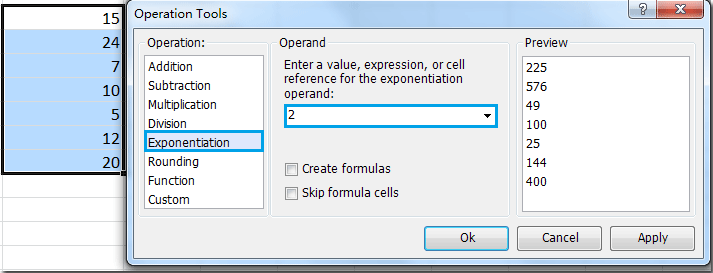2. Then click OK or Apply. The selected cell values have been done the exponentiation at once. See screenshots: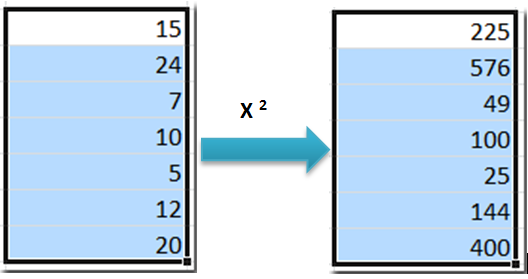####Round a range of numbers to whole numbers or 2 decimal places

Maybe it is easy for you to round a number to a certain decimal place. But if you want to quickly round a range of numbers to whole numbers or 2 decimal places without using formula in Excel, how can you get it done quickly? Such as round 23.456 to 2 decimal places as 23.46 without using formula.

Round a range of numbers to whole numbers

Supposing you have a range numbers that you need to round to the nearest hundred. For example, you want to round the number 5432.123 to the nearest hundred 5400. Please do as follows:

1. Select the range numbers that you want to round. Go to the Operation Tools dialog box, and click Rounding from left Operation Pane. Then enter the number -2 into the Operand box. And you can preview the results from the Preview Pane. See screenshot: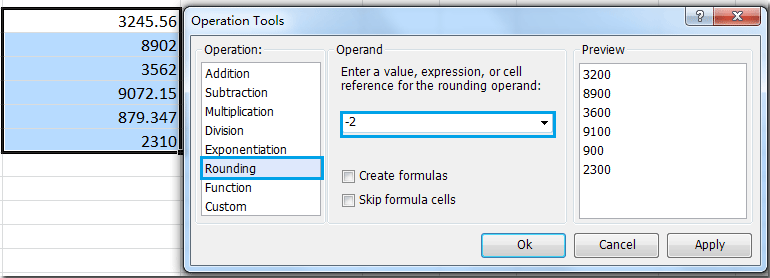2. Then click OK or Apply. The selected cell numbers have been rounded to the nearest 100. See screenshots: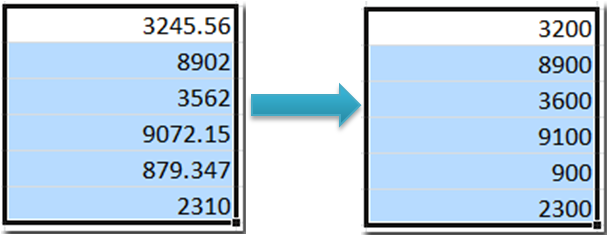Tip: If you want to round the numbers to nearest 10,100, 1000 …, you can enter the number -1, -2, -3 … into the Operand box.

Round a range of numbers to 2 decimal places

If you want to round a range of numbers to 2 decimal places without using formula in Excel, you can do as this:

1. Select the range numbers that you want to round to 2 decimal places. In the Operation Tools dialog box, click Rounding from left Operation Pane. Then enter the number that you want to round to how many decimal places, in this example please enter 2 into the Operand box. And you can preview the results from the Preview Pane. See screenshot: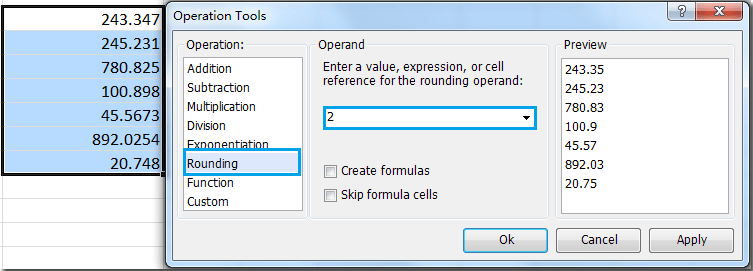2. Then click OK or Apply. The selected cell numbers have been rounded to 2 decimal places. See screenshots: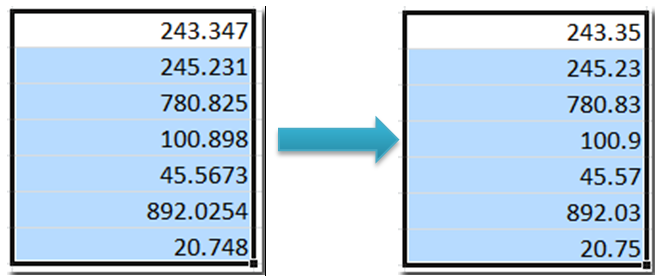####Apply a function to a range of cells

As we all known, there are lots of function in Excel. How do you apply one of the functions to multiple cells and work out the results at once?

1. Select the cells that you want to apply the function. In the Operation Tools dialog box, click Function from left Operation Pane. And then choose one function that you want to apply from the Addition dropdown list. You can preview the results from the Preview Pane. See screenshot: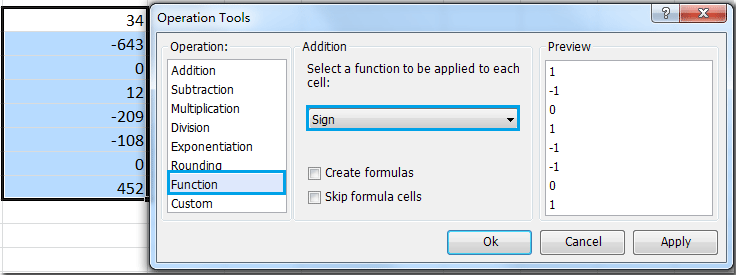2. Then click OK or Apply. This function has been applied to all of the selected cells. See screenshots: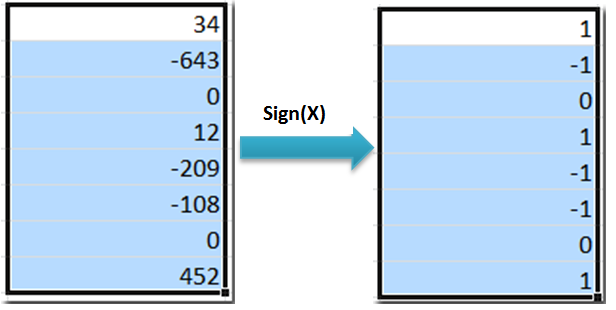####Apply formula to multiple or all cells

Sometimes you need to apply a customized formula to multiple cells in Excel. With this tool, you can quickly apply any formula you need to the cells.

1. Highlight the range that you want to apply the customized formula. Go to the Operation Tools dialog box, click Custom from left Operation Pane. And in the Custom box, input the formula that you need, such as (?*2+5)/5. Tip: The ? symbol represents the value in each cell. You can preview the results from the Preview Pane. See screenshot: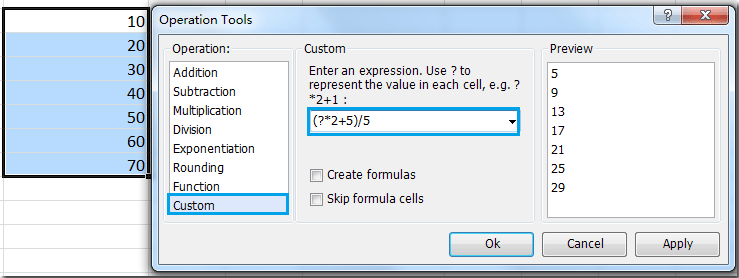2. Then click OK or Apply. The customized formula has been applied to the cells. See screenshots: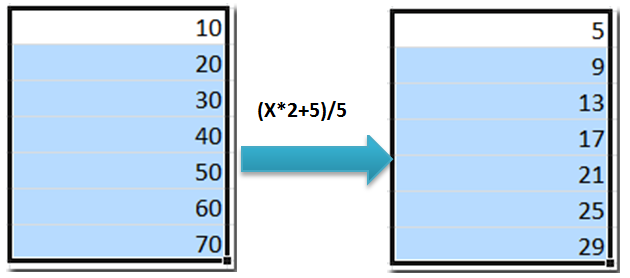Notes:

• If Create formulas option is checked, the results will be in the form of formulas for each cell.
• If Skip formula cells option is checked, the operation will skip and automatically ignore the formula cells in the range.
• You can press Undo (Ctrl+Z) to restore this operation immediately.

Kutools for Excel: with more than 300 handy Excel add-ins, free to try with no limitation in 30 days. Download and free trial Now!

Productivity Tools Recommended
The following tools can greatly save your time and money, which one is right for you?
Office Tab: Using handy tabs in your Office, as the way of Chrome, Firefox and New Internet Explorer.
Kutools for Excel: More than 300 Advanced Functions for Excel 2021, 2019, 2016, 2013, 2010, 2007 and Office 365.

### Kutools for Excel

The functionality described above is just one of 300 powerful functions of Kutools for Excel.

Designed for Excel(Office) 2021, 2019, 2016, 2013, 2010, 2007 and Office 365. Free download and use for 60 days.